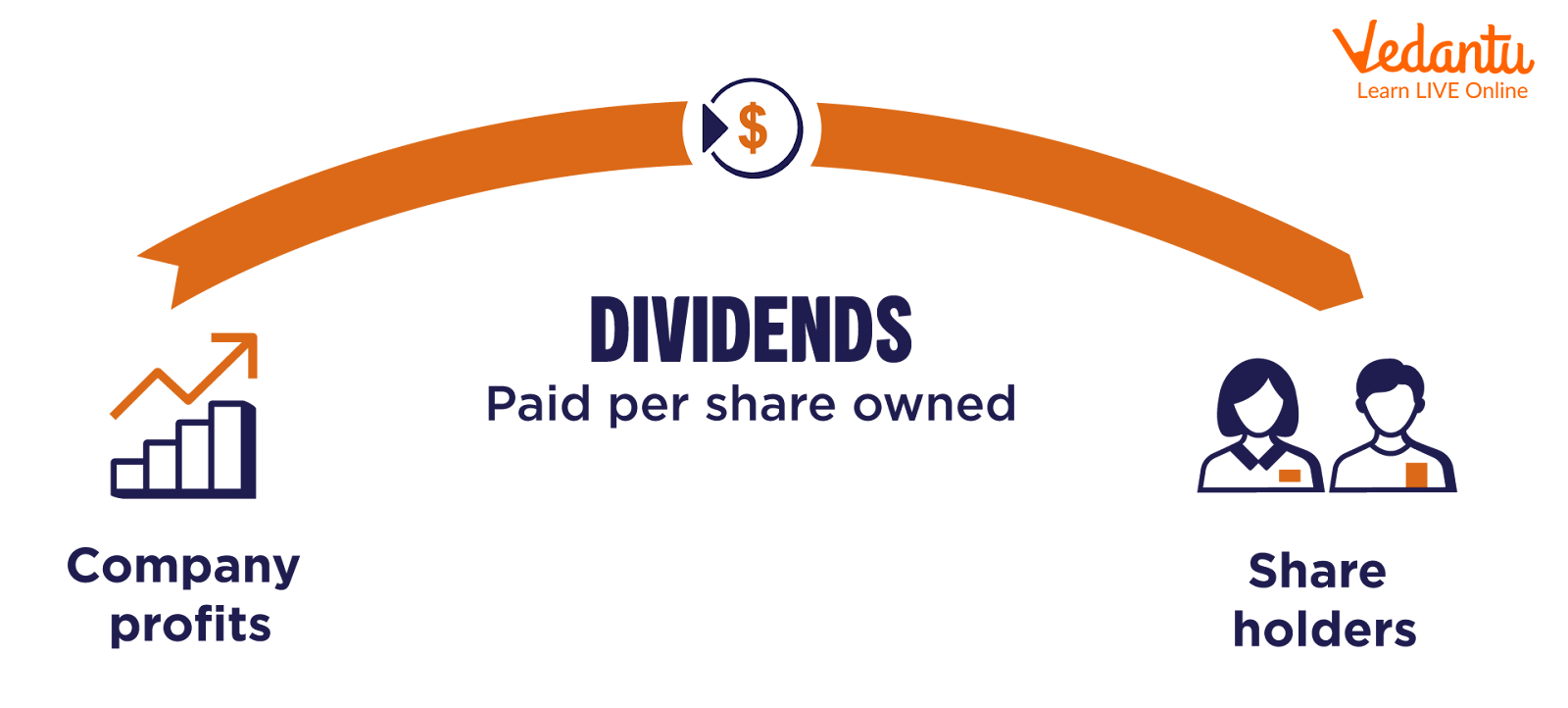Courses
Courses for Kids
Free study material
Free LIVE classes
More

# Rate of Dividend is Calculated On and Formula Explained in Easy WayLIVE
Join Vedantu’s FREE Mastercalss

## Introduction to Rate of Dividend

Dividends are a way for companies to distribute a portion of their profits to their shareholders. The dividend rate measures how much a company's earnings are being paid to shareholders and is often expressed as a percentage. It is an essential metric for investors as it indicates the company's financial health and potential for future growth. The dividend rate is calculated to determine the amount of money a shareholder will receive for each share of stock they own. In this article, we will explain the rate of dividends and its formula in an easy-to-understand way.

## What is a Dividend?

Dividends are amounts that must be divided by other amounts. An algebraic expression, a fraction, or an integer could be used. In algebra, the division is usually represented by placing the dividend over the divisor and separating them with a horizontal line. This horizontal line is also known as a "fraction bar." For example, x divided by y can be written as x/y, which means "x over y" or "divide x by y." In this case, x is the dividend, and y is the divisor.

Take 3 to 4. 3 is the dividend, and 4 is the divisor in this fraction. In fractions, the divisor is referred to as the numerator, and the dividend is the denominator. The outcome of dividing the dividend by a divisor is either an integer or a decimal number.

## Rate of Dividend FormulaFormula of Dividend

The dividend rate is the amount of cash a shareholder gets divided by the value of the shareholder's stock on the market. Divide the annual dividend per share by the stock price to get the dividend rate per share.

Rate of dividend formula = Dividend per share / Price per share at the moment

## Example of Dividend Rate

Let's say a company called "CandyCo" makes and sells candy. They made \$100,000\$ in profits this year. The company decides to give \$20,000\$ of those profits back to their shareholders as dividends. To calculate the dividend rate, we divide the number of dividends paid (\$20,000)\$ by the total profits (\$100,000)\$. So:

\$20,000\$ (dividends) ÷ \$100,000\$ (profits) = 0.2 or 20%

So the dividend rate for CandyCo is 20%. This means that for every dollar of profit, the company makes, 20 cents is paid out to shareholders as dividends.

In a simpler way, you can think of it as a company with a big jar of money (the profits), and they share some of it with the people who own part of the company (the shareholders) as a thank you. The dividend rate is like a "share" of that jar of money they give back to shareholders.

Formula: Dividend Rate = Dividend Per Share / Current Share Price

## Defining Payout Ratio for Dividends

The payout ratio for dividends measures how much of a company's profits are being paid out to shareholders as dividends. It's calculated by dividing the number of dividends paid by the company's earnings per share (EPS).

For example, if a company has an EPS of \$1\$ and pays out \$0.50\$ in dividends, the payout ratio would be 50%. This means that 50% of the company's profits are paid out to shareholders through tips. Another way to think about it is like a company's "sharing plan" or "sharing jar" with its shareholders. The payout ratio tells you what percentage of the company's profits they share with their shareholders as dividends.

A lower payout ratio may indicate that a company is keeping a more significant portion of its profits to reinvest in growth or to build up cash reserves. A higher payout ratio may suggest that the company returns more of its earnings to shareholders.

Formula: Payout ratio for dividends = Total dividends / Net income

## Conclusion

The dividend rate is the annual total projected dividend payments from an investment, fund, or portfolio. It also includes any extra non-recurring dividends that an investor may get during that time. The rate of dividend is fixed or changeable, depending on what the company wants and how it wants to do business. The dividend rate and dividend yield are two terms that are often used interchangeably.

Last updated date: 26th Sep 2023
Total views: 63.3k
Views today: 1.63k

## FAQs on Rate of Dividend is Calculated On and Formula Explained in Easy Way

1. A dividend is calculated on what basis?

For an investment, fund, or portfolio, the dividend rate is calculated by multiplying the most recent dividend payment by the number of dividend payments in a year.

For example, if a fund of investments pays a dividend of 50 cents every three months and an extra dividend of 12 cents per share because of a one-time event that helped the company, the dividend rate is Rs. 2.12 per year (50 cents x 4 quarters + 12 cents = Rs. 2.12).

2. What does dividend rate variance mean?

Companies and industries can have very different dividend rates. For example, investors would probably get higher dividend rates from mature companies in an industry, like basic materials, than from fast-growing technology companies.

Because mature companies don't have many options for investing cash in further growth or capital projects, they give some cashback to their shareholders. A high-growth corporation would employ all available capital to drive its expansion and not pay dividends.

3. What are the signalling effects of dividend rates?

A high dividend rate tells the market two clear and separate things. First, it shows that the company's leaders think the business will be able to generate steady cash flow for the foreseeable future. Second, it shows that management has few options for growth and expansion.